HoldAll, HoldFirst, HoldRest

If a function (f) has the HoldAll attribute (f) does it's work before it's arguments evaluate.
It's easy to see why Clear must have the HoldAll attribute.  Consider the cell where values are assigned to (x,y,z).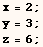If Clear didn't have the HoldAll attribute the following would evaluate to Clear[2,3,6] which is nonsense.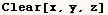The example in the next cell is from Power Programming With Mathematica by David Wagner.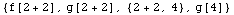In the example above the unevaluated sum (2+2) is substituted for (x) on the right side of the definition  f[x_]:=g[x].  The sum is only evaluated when it's time for (g) to evaluate it's arguments.  If (g) also held it's arguments the sum wouldn't evaluate at all.

The FullForm of (x=2) is Set[x,2].  Set has the HoldFirst attribute and it's important that is does.  If Set didn't hold it's first argument the second assignment in the next cell would evaluate to (2=5) which is nonsense.Also it's easy to see why the symbol If has the attribute HoldRest.  Whe given an expression such as
If[ Test, T, F]  we want to only evaluate 'T' when the Test is True, and we only want to evaluate 'F' when the Test is False.  If the Test is neither True or False we want neither 'T' or 'F' to evaluate.

Built-in Symbols with attributes HoldAll, HoldFirst, HoldRest

The next cell makes a list of all built-in symbols.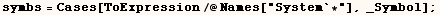The next cell gives a list of all built-in symbols with the HoldAll attribute.The next cell gives a list of all built-in symbols with the HoldFirst attribute.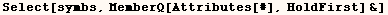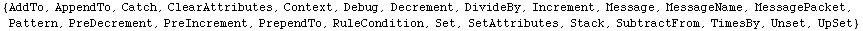The next cell gives a list of all built-in symbols with the HoldRest attribute.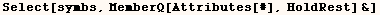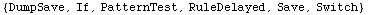Created by Mathematica  (May 16, 2004)

Back to Ted’s Tricks index page